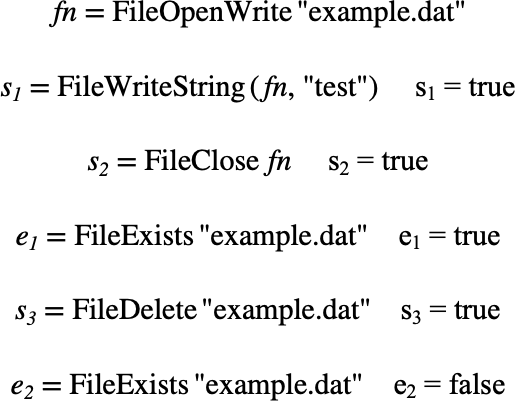# $$\text{FileDelete}$$¶

You can use the $$\text{FileDelete}$$ function to delete a file.

You can use the \filedelete backslash command to insert this function.

The following variants of this function are available:

• $$\text{boolean } \text{FileDelete} \left ( \text{<file number>} \right )$$

• $$\text{boolean } \text{FileDelete} \left ( \text{<filename>} \right )$$

The $$\text{<file number>}$$ is an integer value supplied by the previously called $$\text{FileOpen}$$ function. The $$\text{<filename>}$$ is a text string encoded as a tuple.

The function returns true on success or false if the requested file could not be deleted.

If the function variant accepting a $$\text{<file number>}$$ is used then the opened file will be closed prior to being deleted. You can use the variant accepting a text string on any file.

Figure 133 shows an example use of the $$\text{FileDelete}$$ function.Figure 133 Example Use Of the FileDelete Function